Next: Plane projective transformations Up: No Title Previous: Summary and Properties

# Camera Calibration

The perspective projection from Euclidean 3-space to an image is represented as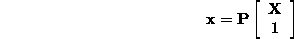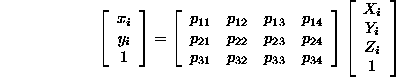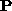is a homogeneous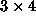camera projection matrix (11 dof) with the decomposition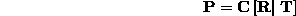The algorithm for camera calibration has two parts:

1. Compute the matrixfrom a set of points with known 3D positions and their measured image positions.
2. Decomposeinto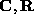and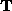via the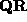decomposition.

1. Compute the matrix: Use correspondences between 3D points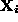and their 2D images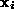to determine the matrix.

1. Each correspondence generates two (linear) equations on the matrix elements of.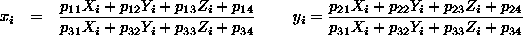multiplying out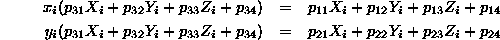2. Given (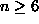) correspondences, a linear solution can be obtained forfrom the set of 2n linear simultaneous equations (cf computation of a projective transformation):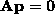, where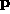is the 12-vector representation of the projection matrix, and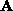is a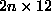matrix. The solution is the eigenvector with least eigenvalue of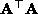.

3. This linear solution is then used as the starting point for a non-linear minimisation of the difference between the measured and projected point: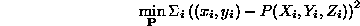Example - Calibration Object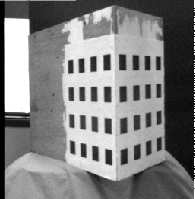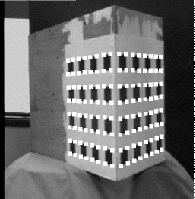Determine accurate corner positions by

1. Extract and link edges using Canny.
2. Fit lines to edges using orthogonal regression.
3. Intersect lines.

The final error between measured and projected points is typically less than 0.02 pixels.

2. Decomposeintoand:

The first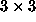submatrix,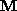, ofis the product (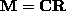) of an upper triangular and rotation matrix.

1. Factorinto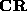using thematrix decomposition. This determines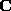and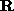.
2. Then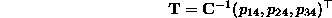Note, this procedure produces a matrix with an extra parameter k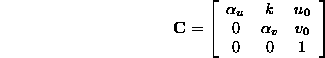with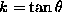, andthe angle between the image axes.Next: Plane projective transformations Up: No Title Previous: Summary and Properties

Bob Fisher
Wed Apr 16 00:58:54 BST 1997